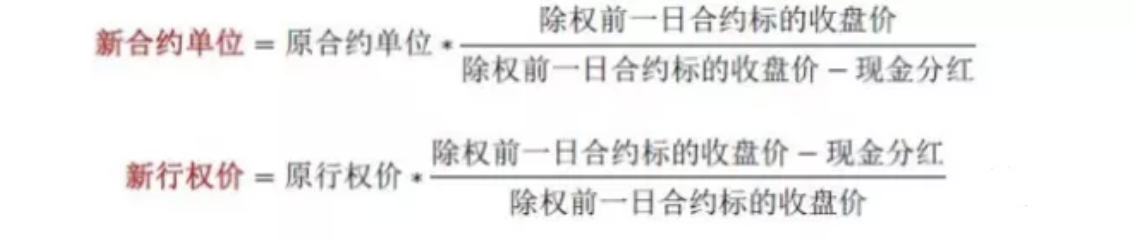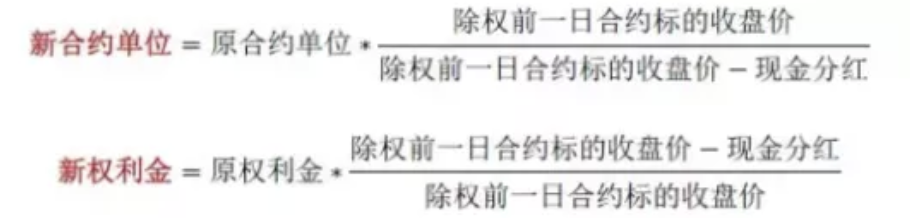FangQuant › Option

### How does the specification of option contract change after 50 ETF dividend?

submitted 2020-01-01 22:05:04

On November 15, 2019, Huaxia Fund Management Co., Ltd. issued the notice on profit distribution of Shanghai Stock Exchange 50 exchange trading open index securities investment fund50ETF. 50ETF will make a dividend of 0.47 yuan / 10 funds, and the ex-dividend date is December 2, 2019. This paper analyzes the impact of this dividend on the option contract specifications. The details are as follows:

(I) contract unit becomes larger and exercise price drops

According to the Shanghai Stock Exchange Stock Option pilot trading rules issued by Shanghai Stock Exchange on January 9, 2015, when the underlying of the contract is ex-right or ex-dividend, the contract unit and exercise price of the option contract shall be adjusted according to the following formula:

New Contract Unit = Old Contract Unit * (1+actual change ratio of floating shares) * underlying closing price before ex-dividend day/[( underlying closing price before ex-dividend day-cash dividend)+price of new issuing * actual change ratio of floating shares]

New execution price* New Contract Unit= Old execution price *Old Contract Unit

This time only involves dividends and nothing else. We can simplify the above formula to get the expression of new contract unit and new exercise price:

New Contract Unit = Old Contract Unit * underlying closing price before ex-dividend day/( underlying closing price before ex-dividend day-cash dividend)

New execution price = Old execution price * (underlying closing price before ex-dividend day-cash dividend)/underlying closing price before ex-dividend day

According to the above formula, it is easy to see that the unit of the contract increase and the exercise price decreases in the later period of ex-dividend.

(II)Decrease of contract price per unit

We use s and K to represent the closing price and option exercise price of 50ETF before dividend, s and K to represent the corresponding data after dividend, and δ to represent the dividend of each unit of fund, then after dividend on December 2, 2019:

S’=S-δ

K’=(S-δ)/S*K

This means that the underlying price and exercise price will change in equal proportion, while the ratio between them will remain unchanged. According to BS formula, we know that:

C=S*N(d1)-K*EXP(-rT)*N(d2)

P=K*EXP(-rT)*N(-d2)-S*N(-d1)

As s and K will change in equal proportion, and D1 and D2 will remain unchanged, after dividend, the price C and P of call and put options shall meet the following requirements:

C’=(S-δ)/S*C

P’=(S-δ)/S*P

That is to say, after dividend, the premium per unit option contract will decrease, and the decrease proportion is equal to the dividend proportion.

(III)The total premium of each contract remains unchanged

According to the above two sections, the new contract unit after dividend is in line with the new unit premium (the premium in the table below refers to the unit premium corresponding to the unit contract):

New Contract Unit = Old Contract Unit * underlying closing price before ex-dividend day/( underlying closing price before ex-dividend day-cash dividend)

New Premium = Old Premium * (underlying closing price before ex-dividend day-cash dividend)/underlying closing price before ex-dividend day

It is not hard to find that the total premium of each contract (contract unit * unit premium) meets:

New Contract Unit * New Premium= Old Contract Unit * Old Premium

This shows that the total premium of each contract will not be changed due to the dividend of the underlying, regardless of the call or put options.

(IV)Listing of new standard contracts

On Dec 2, Shanghai Stock Exchange will re-list the new standard contracts for each month according to the ex-right and ex-dividend price after 50ETF dividend. The new contracts include two types: call and put, four expiration months (December 2019, January 2020, March 2020 and June), nine exercise prices (one equal value, four real values and four virtual values), a total of 72 contracts. The new contract units are all 10000.

50ETF分红后，期权合约规格如何变？
2019年11月15日，华夏基金管理有限公司发布《上证50交易型开放式指数证券投资基金利润分配公告》，上证50交易型开放式指数证券投资基金（证券简称为“50ETF”，证券代码为“510050”）将进行0.47元/10份基金的分红，除息日为2019年12月2日。本文对本次分红对期权合约规格造成的影响进行了分析。具体如下：
（一）合约单位变大、行权价下降（二）每单位合约权利金下降（三）每张合约总权利金不变（四）挂牌新的标准合约
12月2日，上交所将按照50ETF分红后除权除息价格重新挂牌新的各个月份标准合约，新挂合约包括认购、认沽两种类型，四个到期月份（2019年12月、2020年1月、3月和6月），九个行权价格（1个平值、4个实值、4个虚值），共72个合约。新挂合约的合约单位均为10000。